NEET  >  Test: Physics - 11

# Test: Physics - 11

Test Description

## 45 Questions MCQ Test NEET Mock Test Series | Test: Physics - 11

Test: Physics - 11 for NEET 2023 is part of NEET Mock Test Series preparation. The Test: Physics - 11 questions and answers have been prepared according to the NEET exam syllabus.The Test: Physics - 11 MCQs are made for NEET 2023 Exam. Find important definitions, questions, notes, meanings, examples, exercises, MCQs and online tests for Test: Physics - 11 below.
Solutions of Test: Physics - 11 questions in English are available as part of our NEET Mock Test Series for NEET & Test: Physics - 11 solutions in Hindi for NEET Mock Test Series course. Download more important topics, notes, lectures and mock test series for NEET Exam by signing up for free. Attempt Test: Physics - 11 | 45 questions in 45 minutes | Mock test for NEET preparation | Free important questions MCQ to study NEET Mock Test Series for NEET Exam | Download free PDF with solutions
 1 Crore+ students have signed up on EduRev. Have you?
Test: Physics - 11 - Question 1

### The acceleration of moon with respect to earth is 0.0027m/s2  and the acceleration of an apple falling on earth’s surface is about 10m/s2 .Assume that the radius of the moon is one fourth of the earth’s radius. If the moon is stopped for an instant and then released, it will towards the earth. The initial acceleration of the moon towards the earth will be

Test: Physics - 11 - Question 2

### The acceleration of moon with respect to earth is 0.0027m/s2  and the acceleration of an apple falling on earth’s surface is about 10m/s2 .Assume that the radius of the moon is one fourth of the earth’s radius. If the moon is stopped for an instant and then released, it will towards the earth.  The acceleration of the moon just before it strikes the earth-

Test: Physics - 11 - Question 3

### A person brings a mass of 1 kg from infinity to a point A. Initially the mass was at rest but it moves at a speed of 2m/s as it reaches A. The work done by the person on the mass is –3J. The potential at A is

Test: Physics - 11 - Question 4
The time period of an earth-satellite in circular orbit is independent of
Test: Physics - 11 - Question 5

Figure shown the elliptical path of a planet about the sun. The two shaded parts have equal area. If tand tbe the time taken by the planet to go from a to b and from c to d respectively,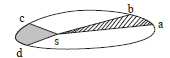Test: Physics - 11 - Question 6

A particle is kept at rest at a distance R(earth’s radius) above the earth’s surface. The minimum speed with which it should be projected so that it does not return is

Detailed Solution for Test: Physics - 11 - Question 6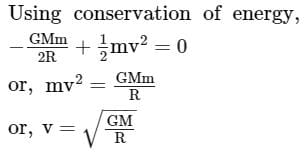Test: Physics - 11 - Question 7
A uniform spherical shell gradually shrinks maintaining its shape. The gravitational potential at the center
Test: Physics - 11 - Question 8

Two satellites A and B move round the earth in the same orbit. The mass of B is twice the mass of A

Test: Physics - 11 - Question 9
The motion of a particle is given by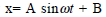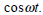The motion of the particle is
Test: Physics - 11 - Question 10
A particle executes simple harmonic motion with a frequency v. The frequency with which the kinetic
energy oscillates is
Test: Physics - 11 - Question 11

A particle executes simple harmonic motion under the restoring force provided by a spring. The time period is T. If the spring is divided in two equal parts and one part is used to continue the simple harmonic motion , the time period will

Test: Physics - 11 - Question 12

The free end of a simple pendulum is attached to the ceiling of a box. The box is taken to a height and the point, the box is released to fall freely. As seen from the box during this period, the bob will

Test: Physics - 11 - Question 13
A particale moves in a circular path with a uniform speed. Its motion is
Test: Physics - 11 - Question 14
Which of the following quantities are always zero in a simple harmonic motion ?
Test: Physics - 11 - Question 15

A particle moves on the x-axis according to the equation x = x0 sin2ωt. The motion is simple harmonic

Test: Physics - 11 - Question 16

A sine wave is travelling in a medium. A particular particle has zero displacement at a certain instant. The particle closest to it having zero displacement is at a distance

Test: Physics - 11 - Question 17

Both the strings, shown in figure are made of same material and have same cross-section. The pulleys are light. The wave speed of a transverse wave in the string AB is vand in CD it is v2. Then v1/v2 is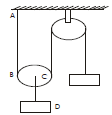Test: Physics - 11 - Question 18

A wave pulse, travelling on a two-piece string, gets partially reflected and Partially transmitted at the Junction. The reflected wave is inverted in shape as compared to the incident one. If the incident wave has wavelength λ and the transmitted wave λ

Test: Physics - 11 - Question 19

Two waves represented by y = a sin (ωt-kx) and y = a cos(ωt-kx)  are superposed. The resultant wave will have an amplitude

Test: Physics - 11 - Question 20

Consider two waves passing through the same string.Principle of superposition for displacement says that the net displacement of a particle on the string is sum of the displacements proudced by the two waves individually. Suppose we state similar principles for the net velocity of the particle and the kinetic energy of the particle. Such a principle will be valid for

Test: Physics - 11 - Question 21

Two sine waves travel in the same direction in a medium.The amplitude of each wave is A and the phase difference between the two waves is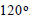The resultant amplitude will be

Test: Physics - 11 - Question 22

A sonometer wire of length l vibrates in fundamental mode when excited by a tuning fork of frequency 416Hz. If the length is doubled keeping other things same,the string will

Test: Physics - 11 - Question 23
Longitudinal waves cannot
Test: Physics - 11 - Question 24

Consider the following statements about sound passing through a gas
A. The pressure of the gas at a point oscillates in time
B. The position of a small layer of the gas oscillates in time

Test: Physics - 11 - Question 25

A tuning fork sends sound waves in air. If the temperature of the air increases, which of the following parameters will change ?

Test: Physics - 11 - Question 26

The speed of sound in a medium depends on

Test: Physics - 11 - Question 27
An organ pipe, open at both ends, contains
Test: Physics - 11 - Question 28
A cylindrical tube, open at both ends, has a fundamental frequency v. The tube is dipped vertically in water so that half of its length is inside the water. The new fundamental frequency is
Test: Physics - 11 - Question 29

A tuning fork of frequency 512 Hz is vibrated with a sonometer wire and 6 beats per second are heard. The beat frequency reduces if the tension in the string is slightly increased. The original frequency of vibration of the string is

Detailed Solution for Test: Physics - 11 - Question 29

f1 = 512Hz
f2 = frequency of sonometer wire.
Now, 512 -f2 = 6
By increasing the tension in sonometer wire fwill increase.
In first case, beat frequency will decreae.
∴ 512 - f2 = 6
or f2 = 506 Hz

Test: Physics - 11 - Question 30

A small sources of sound moves on a circle as shown in figure and an observer is sitting at O.Let  v1,v2,vbe the frequencies heard when the source is at A,B and C respectively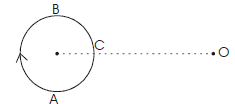Test: Physics - 11 - Question 31
When you speak to your friend, which of the following parameters have a unique value in the sound produced?
Test: Physics - 11 - Question 32

The fundamental frequency of a vibrating organ pipe is 200 Hz

Test: Physics - 11 - Question 33

A simple pendulum with a brass bob has a time period T. The bob is now immersed in a non-viscous liquid and oscillated. If the density of the liquid is 1/8 that of brass, the time period of the same pendulum will be

Detailed Solution for Test: Physics - 11 - Question 33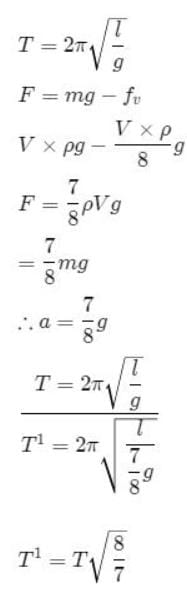Test: Physics - 11 - Question 34
In the figure, the block of mass m, attached to the spring of stiffness k is in contact with the completely elastic wall, and the compression in the spring is e.The spring is compressed further by e by displacing the block towards left and is, then released. If the collision between the block and the wall is completely elastic, then the time period of oscillations of the block will be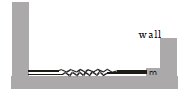Test: Physics - 11 - Question 35

Two sounding bodies producing progressive waves given by y1 = 4sin(400πt) and y2 = 3sin(404πt) where t is in seconds, are situated near the ears of a person. The person will hear

Test: Physics - 11 - Question 36

A closed organ pipe of length L and an open organ contain gases of densities ρ1 and ρrespectively.The compressibility of gases are equal in both the pipes. Both the pipes are vibrating in their first overtone with same frequency. The length of the organ pipe is

Test: Physics - 11 - Question 37

A wire of 9.8 x 10-3kgm-1 passes over a frinctionless light pulley fixed on the top of frictionless inclined plane which makes an angle of 30with the horizontal. Masses m and M are tied at the two ends of wire such that m rests on the plane and M hangs freely vertically downwards. The entire system is in equilibrium and a transverse wave propagates along the wire with a velocity of 100 ms-1.Choose the correct option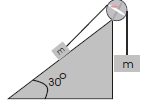Test: Physics - 11 - Question 38
Direction : Read the following question and choose
A. If both Assertion and Reason are correct and reason is the correct explanation of assertion
B. If both Assertion and Reason are true, but Reason is not correct explanation of the Assertion
C. If Assertion is true, but the Reason is false
D. If Assertion is false, but the Reason is true
E. If both assertion and reason are false
Assertion : Compression and rarefaction involve changes in density and pressure
Reason : When particles are compressed, density of medium increases and when they are rerefied, density of medium decrease
Test: Physics - 11 - Question 39
Direction : Read the following question and choose
A. If both Assertion and Reason are correct and reason is the correct explanation of assertion
B. If both Assertion and Reason are true, but Reason is not correct explanation of the Assertion
C. If Assertion is true, but the Reason is false
D. If Assertion is false, but the Reason is true
E. If both assertion and reason are false
Assertion : On a rainy day sound travel slower than on a dry day
Reason : When moisture is present in air the density of air increases
Test: Physics - 11 - Question 40
Direction : Read the following question and choose
A. If both Assertion and Reason are correct and reason is the correct explanation of assertion
B. If both Assertion and Reason are true, but Reason is not correct explanation of the Assertion
C. If Assertion is true, but the Reason is false
D. If Assertion is false, but the Reason is true
E. If both assertion and reason are false
Assertion : An open organ pipe of certain length have the same fundamental frequency as closed organ pipe of half the length
Reason : In the case of open oran pipe, at both the ends antinodes are formed, while in the closed organ pipe at one end antinode and at the other node is formed
Test: Physics - 11 - Question 41
Direction : Read the following question and choose
A. If both Assertion and Reason are correct and reason is the correct explanation of assertion
B. If both Assertion and Reason are true, but Reason is not correct explanation of the Assertion
C. If Assertion is true, but the Reason is false
D. If Assertion is false, but the Reason is true
E. If both assertion and reason are false
Assertion : We can recognise our friends by listening their voices
Reason : The quality of sound produced by different persons are different
Test: Physics - 11 - Question 42
Direction : Read the following question and choose
A. If both Assertion and Reason are correct and reason is the correct explanation of assertion
B. If both Assertion and Reason are true, but Reason is not correct explanation of the Assertion
C. If Assertion is true, but the Reason is false
D. If Assertion is false, but the Reason is true
E. If both assertion and reason are false
Assertion : In simple harmonic total mechanical energy can be negative also
Reason : Potential energy is always negative and if it is greater than kinetic energy total mechanical energy will be negative
Test: Physics - 11 - Question 43
Direction : Read the following question and choose
A. If both Assertion and Reason are correct and reason is the correct explanation of assertion
B. If both Assertion and Reason are true, but Reason is not correct explanation of the Assertion
C. If Assertion is true, but the Reason is false
D. If Assertion is false, but the Reason is true
E. If both assertion and reason are false
Assertion : In SHM let x be the maximum speed, y the frequency of oscillation and z the maximum acceleration then xy /z is a constant quanitity
Reason : The is because xy /z becomes a dimensionless quantiy
Test: Physics - 11 - Question 44
Direction : Read the following question and choose
A. If both Assertion and Reason are correct and reason is the correct explanation of assertion
B. If both Assertion and Reason are true, but Reason is not correct explanation of the Assertion
C. If Assertion is true, but the Reason is false
D. If Assertion is false, but the Reason is true
E. If both assertion and reason are false
Assertion : At height h from ground and at depth h below ground, where h is approximately equal to 0.62 R, the value of g acceleration due to gravity is same
Reason : Value of g decreases both sides, in going up and down
Test: Physics - 11 - Question 45
Direction : Read the following question and choose
A. If both Assertion and Reason are correct and reason is the correct explanation of assertion
B. If both Assertion and Reason are true, but Reason is not correct explanation of the Assertion
C. If Assertion is true, but the Reason is false
D. If Assertion is false, but the Reason is true
E. If both assertion and reason are false
Assertion : For a system of masses, at some finite distance gravitational field can be zero but gravitational potential cannot
Reason : Gravitational field is a vector quantity, while potential a scalar one

## NEET Mock Test Series

1 videos|25 docs|144 tests
 Use Code STAYHOME200 and get INR 200 additional OFF Use Coupon Code
Information about Test: Physics - 11 Page
In this test you can find the Exam questions for Test: Physics - 11 solved & explained in the simplest way possible. Besides giving Questions and answers for Test: Physics - 11, EduRev gives you an ample number of Online tests for practice

## NEET Mock Test Series

1 videos|25 docs|144 tests

### How to Prepare for NEET

Read our guide to prepare for NEET which is created by Toppers & the best Teachers